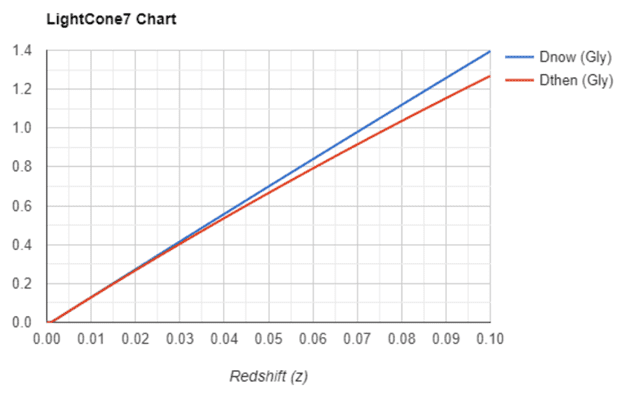# Edwin Hubble and the Evidence for Universe Expansion

• I
Gold Member
If you want the same set of objects with modern distance/velocity values, you can plot it yourself. Use the tables in that paper to find each object in any public database.
Hi @Bandersnatch:

I will do the plot myself you recommend if I need to. I would prefer to not have to do this. What would help me is help with reading the 2 X labels and the 2 Y labels:
The X labels seem to be 106 parsecs and 2 x 106 parsecs. Is that correct? The Y labels are more difficult to read.

Regards,
Buzz

labels
x: 1 and 2 Mpc
y: 500 and 1000 km/s

•Buzz Bloom
Gold Member
I will do the plot myself you recommend if I need to. I would prefer to not have to do this. What would help me is help with reading the 2 X labels and the 2 Y labels:

The X labels seem to be 106 parsecs and 2 x 106 parsecs. Is that correct? The Y labels are more difficult to read.

I plotted the v vs. r data shown in Table 1 of Hubble’s 1929 paper and compared it with his Fig. 1. I noticed that some of the data points are not placed correctly in his figure. This does not change things; the Hubble constant determined from his figure, about 500 km/s/Mpc, is too high anyway.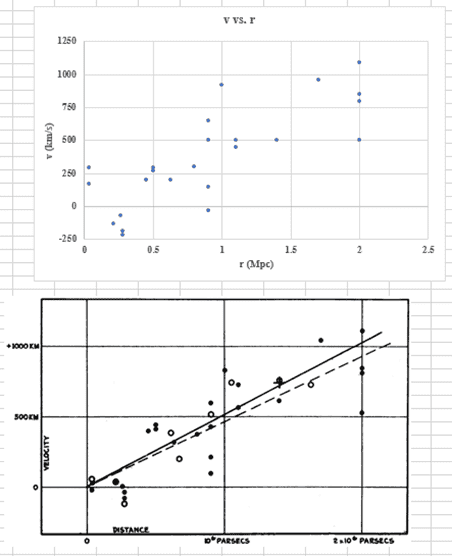•Buzz Bloom
(...) Fig. 1. I noticed that some of the data points are not placed correctly in his figure.
Those data need to be corrected for solar motion, as discussed in the paper. You can see when comparing the two graphs that the differences are on the order of +/- 200 km/s.

In any case, I think Buzz wanted to plot the original set of galaxies but with modern data for their distances and velocities.

•Buzz Bloom
Gold Member
Those data need to be corrected for solar motion, as discussed in the paper. You can see when comparing the two graphs that the differences are on the order of +/- 200 km/s.

If not in Table 1 and Table 2, where in the paper are the plotted, corrected v vs. r data?

If not in Table 1 and Table 2, where in the paper are the plotted, corrected v vs. r data?
It's not given explicitly in the paper. There is the raw data in table 1, values for solar motion are extracted (under the first equation), and the corrected data plotted.

Gold Member
It's not given explicitly in the paper. There is the raw data in table 1, values for solar motion are extracted (under the first equation), and the corrected data plotted.

I am confused. Can you calculate for one case to demonstrate the use of the following equation? Do we know the angles needed?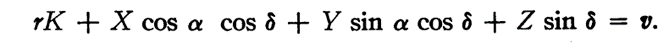Gold Member
It's not given explicitly in the paper. There is the raw data in table 1, values for solar motion are extracted (under the first equation), and the corrected data plotted.

Referring to Table 1 in his paper, Hubble writes,

The data in the table indicate a linear correlation between distances and velocities, whether the latter are used directly or corrected for solar motion, according to the older solutions.​

As you said, the raw v vs. r data are listed in Table 1. However, the corrected data are used in Fig. 1. The methods of correction are discussed in the paper, but the results are not listed in it.

This explains the discrepancy shown in Post #38.

I went and graphed Hubble's table 1 data corrected for his assessment of solar motion (individual objects only):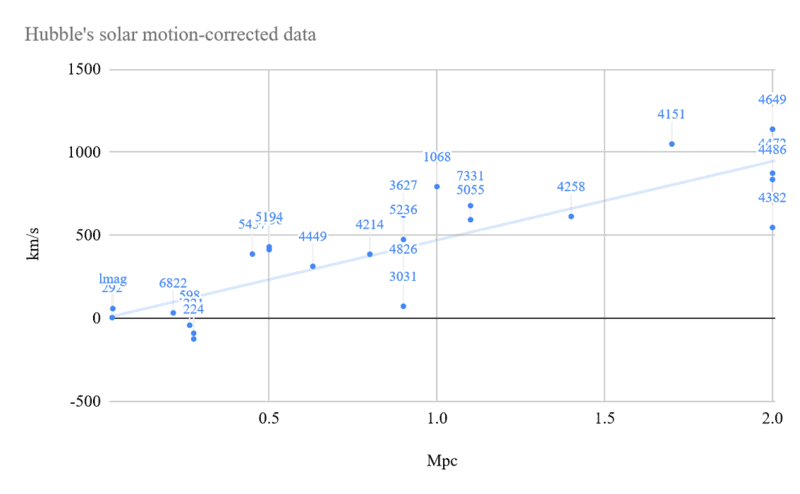Looks almost the same as the graph in the paper. He did mention also adding residuals to the final graph, so maybe that's where the remaining differences are. But I don't know how it's done.
Took me way too long to figure out how to remove the solar motion correctly (hopefully), since apparently I completely forgot how to work in spherical coordinates.

For comparison, here's the same graph using modern data (from Cosmicflows-3 & 1) for the same set of objects: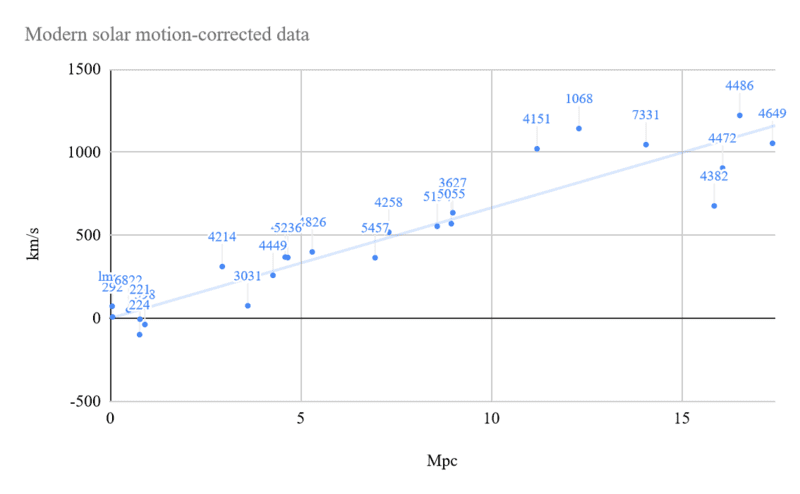The order of magnitude underestimation of distances in the original work is immediately apparent.

Here's a link to the spreadsheet, in case anyone wants to play with it (you'll have to make a copy to edit).

Gold Member
I went and graphed Hubble's table 1 data corrected for his assessment of solar motion . . .

For comparison, here's the same graph using modern data (from Cosmicflows-3 & 1) for the same set of objects . . .

That’s very impressive!

I put the original data from Hubble’s Table 1 and your corrected data in one figure: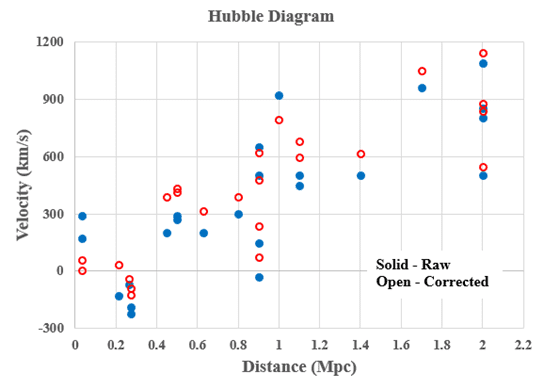The Hubble constant estimated from your graph using modern data is about 69.4 km/s/Mpc.

Just a note, I replaced the values for right ascension, declination, and solar velocity that I thought Hubble was using (the rounded average between the two solutions) with values he derived from the 24 objects only, and the graph now looks identical to what's in the paper. As far as I can tell, at least.
Only the trend line better matches the solution for groups of objects, for some reason.

If anyone knows what is meant by adding residuals to the solution, which doesn't seem to have any visible effect anyway - do tell. I thought he meant uncertainties, but there's no error bars on the graph, nor can I see any way in which they'd 'average 150 and 100 km/s'.

Gold Member
For comparison, here's the same graph using modern data (from Cosmicflows-3 & 1) for the same set of objects:
View attachment 296757

I have a question about the distances used in the graph. Are they the distances at emissions of the received photons or the present distances of the galaxies? They can be quite different at higher z values (e.g., z = 0.1).

I have a question about the distances used in the graph. Are they the distances at emissions of the received photons or the present distances of the galaxies? They can be quite different at higher z values (e.g., z = 0.1).
With these objects you don't even get to z=0.01, so which distance is reported makes no appreciable difference. Especially seeing how actual error bars on the measurements have been omitted. This database reports either comoving or luminosity distance - I'd have to check. But, again, this close, and at this level of imprecision, it doesn't matter much which one it is. They're all approximately equal.

Gold Member
With these objects you don't even get to z=0.01, so which distance is reported makes no appreciable difference.

If we are only talking about Hubble’s 1929 paper, I agree with you. However, in the reference you gave in Post #12, the distances extend to about 400 Mpc (z values extend to about 0.1). As can be seen from the output of Jorrie’s calculator, the difference can be significant: